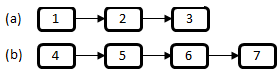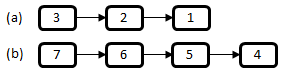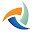## Monday, February 18, 2013

### No. 40 - Add on Lists

Problem: Nodes in a list represent a number. For example, the nodes in Figure 1 (a) and (b) represent numbers 123 and 4567 respectively. Please implement a function/method to add numbers in two lists, and store the sum into a new list.Figure 1: Two lists representing numbers. (a) A list for 123; (b) A list for 4567.
Analysis: Usually numbers are added beginning from the least significant digits (The digit 3 in the number 123, and the digit 7 in the number 4567). As shown in Figure 1, the least significant digits are at the tail of lists, and they can be accessed after the whole lists are scanned. Therefore, lists should be reversed at first, in order get the least significant digits before other digits. The two reversed lists of lists in Figure 1 are shown in Figure 2.Figure 2: Two reversed lists of the lists in Figure 1.

After two lists are reversed, we can add nodes along the links between nodes, and then reversed the result list after all nodes are added. Therefore, the overall structure to add numbers in two lists can be implemented with the following code in C/C++:

{
return NULL;

return Reverse(pResult);
}

Now let’s implement the function AddReversed, to add nodes in two reversed lists. Digits are gotten in nodes along links between nodes. When we get two digits in two lists, we add them and create a new node to store the sum, and append the new node into the list for result. There are two issues worthy of attention: (1) The length of two lists might be different; (2) The sum of two digits may be greater than 10, so we have to take care of the carry when adding two digits. The function AddReversed can be implemented with the following C/C++ code:

{
int carry = 0;
ListNode* pPrev = NULL;
{

}
if(carry > 0)
{
ListNode* pNode = CreateListNode(carry);
}

}

The function AddNode adds digits in two nodes. The third parameter of this function takes the carry for addition calculation, as listed below:

ListNode* AddNode(ListNode* pNode1, ListNode* pNode2, int* carry)
{
int num1 = 0;
if(pNode1 != NULL)
num1 = pNode1->m_nValue;
int num2 = 0;
if(pNode2 != NULL)
num2 = pNode2->m_nValue;

int sum = num1 + num2 + *carry;
*carry = (sum >= 10) ? 1 : 0;

int value = (sum >= 10) ? (sum - 10) : sum;
return CreateListNode(value);
}

The function AppendNode is used append a node into the tail of lists. In order to avoid scanning the whole list to get the previous tail every time, the previous tails is stored in the parameter/variable pPrev, as listed in the following code:

void AppendNode(ListNode** pHead, ListNode** pPrev, ListNode* pNode)
{
if(*pPrev == NULL)
*pPrev = pNode;
else
{
(*pPrev)->m_pNext = pNode;
*pPrev = pNode;
}
}

The function CreateListNode is to create a list node according to a value, which is omitted here because it’s quite straightforward.

The steps to reverse a list are discussed in my previous blog.

More coding interview questions are discussed in my book <Coding Interviews: Questions, Analysis & Solutions>. You may find the details of this book on Amazon.com, or Apress.

The author Harry He owns all the rights of this post. If you are going to use part of or the whole of this ariticle in your blog or webpages, please add a reference to

1.Hi there, I think this is a good solution. However.. If I were you I would actually avoid bothering with carrying the digits. The way to handle that is to convert each of the two lists into numbers and then add them. So you can have something like:

int result = 0;
int multiplier = 1;
while(revPtr) {
result = result + (revPtr->value * multiplier);
multiplier = multiplier * 10;
revPtr = revPtr->next;
}
return result;
}

Now that you can convert a list into a number, just go ahead and write a wrapper function to add them ;)

int sumLists(Node* x, Node* y) {
return generateNumberFromList(x) + generateNumberFromList(y);
}

This way you can easily implement any numeric operation between numbers implemented as lists .. With your current approach addition is fairly easy to knock out.. but go ahead and try division... that would be quite error prone..

Anyway, just my to cents. Btw I really enjoy your blog and just got your book as well, nice work ! I like the questions because they are jus the right level of complexity. Keep it up !

1.oh dear... is my formatting bad or what....

2.The problem is if the numbers are very large. What if you had lists with 100 entries? or 1000 entries?

The point is that this should work with lists or arbitrary length.

2.Why to do all the reverse and sum each digits and the maintain the carry for each iteration.?

Why don't you simply traverse a list and make the number like 123 and from other list make number 4567

simply add these number as integer and extract each number from result and make another list

1.Your solution is quick and easy, but what happens if the linked list represents a number bigger than what an int/long/double can hold?
Your solution won't work anymore, and this is what the interviewer is really testing you for.

3.I think it would be better to use a stack and add the digits on top

4.This comment has been removed by the author.

5.This comment has been removed by the author.

6.This comment has been removed by the author.

7.It makes sense to reverse the operand-lists for reasons best known. However, we might want to still consider the possibility of getting away with the idea of reversing the final resultant list. That list is now synthesized here by inserting the running result node at the head-end instead of where it is done hitherto. Evenutally, the head will naturally point to the highest place value. Having said that, illustrating with the very same example cited in this blog -

List - A: 1->2->3
List - B: 4->5->6->7

Now ordering the lists (exactly as per the preferred original design) -
List - A: 3->2->1
List - B: 7->6->5->4

Synthesizing Resultant list
---------------------------
Head initialized to NULL, Carry to 0
Carry = 0; Resultant_list->NULL

Now, lets start pushing the results one by one on to the Resultant_list:
List - A: 3->2->1
List - B: 7->6->5->4->0

Resultant_list->0->NULL
List - A: 2->1
List - B: 6->5->4->0
Carry = 1

Resultant_list->9->0->NULL
List - A: 1
List - B: 5->4->0
Carry = 0

Resultant_list->9->0->NULL
List - A: 1
List - B: 5->4->0
Carry = 0

Resultant_list->6->9->0->NULL
List - A: NULL
List - B: 4->0
Carry = 0

Resultant_list->4->6->9->0->NULL
List - A: NULL
List - B: 4->0
Carry = 0

So in effect, we have actually done away with the final reversal that was needed earlier; note that the Resultant_list is already formed in right order. And now, with that philosophy, lets turn back at the original code and get it simplified as under -

{
return NULL;

}

{
int carry = 0;

{

}

if(carry > 0)
{
ListNode* pNode = CreateListNode(carry);
}

}

ListNode* AddNode(ListNode* pNode1, ListNode* pNode2, int* carry)
{
int balance = *carry;
if (pNode1 != NULL) balance += pNode1->m_nValue;
if (pNode2 != NULL) balance += pNode2->m_nValue;
if (balance >= 10) {
balance -= 10;
*carry = 1;
} else *carry = 0;

return CreateListNode(balance);
}

{
if (!pNode) return;
}

Well cool, that has relaxed us from reversing the result list as well as the headache of the additional node - pPrev that was maintained hitherto, further simplifying the amalgam of code at large.

8.The stated question doesn't make sense. What are you trying to do? Add all the nodes? Sum the nodes with corresponding indices?

9.Instead of appending nodes + final reversal of third list, try prepending nodes to make third list (no reversal needed then).

Also, a littler simpler to prepend not append :)

10.